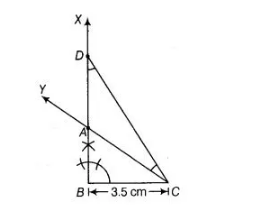# A right triangle when one side is 3.5 cm`
Question:

A right triangle when one side is 3.5 cm and sum of other sides and the hypotenuse is 5.5 cm.

Solution:

Let given right triangle be $\mathrm{ABC}$.

Then, given $B C=3.5 \mathrm{~cm}, \angle B=90^{\circ}$ and sum of other side and hypotenuse i.e., $A B+A C=5.5 \mathrm{~cm}$ To construct $\triangle \mathrm{ABC}$ use the following steps

1. Draw the base $B C=3.5 \mathrm{~cm}$

2. Make an angle $X B C=90^{\circ}$ at the point $B$ of base $B C$.3. Cut the line segment BD equal to AB + AC i.e., $5.5 \mathrm{~cm}$ from the ray XB.

4. Join $D C$ and make an $\angle D C Y$ equal to $\angle B D C$.

5. Let $Y$ intersect $B X$ at $A$.

Thus, $\triangle \mathrm{ABC}$ is the required triangle.

Justification

Base $B C$ and $\angle B$ are drawn as given.

In $\triangle A C D, \angle A C D=\angle A D C$ [by construction]

$A D=A C$...(i)

[sides opposite to equal angles are equal] Now, $A B=B D-A D=B D-A C$ [from Eq. (i)]

$=>B D=A B+A C$

Thus, our construction is justified.